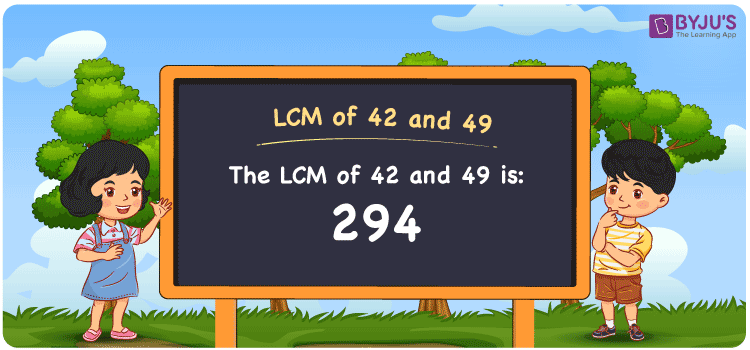# LCM of 42 and 49

LCM of 42 and 49 is 294. LCM stands for the Least Common Multiple or Lowest Common Multiple. LCM is the smallest numeral that is divisible by the given group of numbers. In the given set of numbers 42 and 49, 294 is the first(least or smallest) number that is common in the set of common multiples of 42 and 49. The LCM which is also known as LCD, Least Common Divisor for 42 and 49 is 294. You can read more on LCM for further details.

## What is LCM of 42 and 49

The Least Common Multiple or Lowest Common Multiple of 42 and 49 is 294.## How to Find LCM of 42 and 49?

LCM of 42 and 49 can be determined using three methods:

• Prime Factorisation
• Division method
• Listing the multiples

### LCM of 42 and 49 Using Prime Factorisation Method

In the Prime Factorisation method, the numbers 42 and 49 can be expressed as;

42 = 2 × 3 × 7

49 = 7 × 7

7 is the common factor for 42 and 49. 7 is multiplied with other factors of 42 and 49 to get the LCM.

LCM (42, 49) = 2 × 3 × 7 × 7 = 294

### LCM of 42 and 49 Using Division Method

In the Division Method, the given set of numbers 42 and 49 are divided by prime divisors together. The product of prime divisors is the LCM.

 2 42 49 3 21 49 7 7 49 7 1 7 × 1 1

Therefore LCM (42, 49) = 2 × 3 × 7 × 7 = 294

### LCM of 42 and 49 Using Listing the Multiples

By listing all the multiples of 42 and 49, we can identify the LCM. Below is the list of multiples for 42 and 49

 Multiples of 42 Multiples of 49 42 49 84 98 126 147 168 196 210 245 252 294 294 343

LCM (42, 49) = 294

## Video Lesson on Applications of LCM## Solved Examples

What is the smallest number that is divisible by both 42 and 49?

Answer: 294 is the smallest number that is divisible by both 42 and 49.

What is the LCM for 7, 42 and 49?

Answer: LCM for 7, 42 and 49 is 294 as 7 is the factor of 42 and 49.

## Frequently Asked Questions on LCM of 42 and 49

### What is the LCM of 42 and 49?

The LCM of 42 and 49 is 294.

### Is the LCM of 42 and 49 the same as the Highest Common Factor of 42 and 49?

No. The Least Common Multiple of 42 and 49 is 294 and the Highest Common Factor of 42 and 49 is 7.

### What are the common factors of 42 and 49?

1 and 7 are the common factors of 42 and 49.

### What are the first 3 common multiples of 42 and 49?

The first three common multiples of 42 and 49 are 294, 588, and 882.

### Is the LCM of 6 and 42 the same as the LCM of 6 and 49?

No. LCM of 6 and 42 is 42, whereas the LCM of 6 and 49 is 294. They are not the same.